# Calculator

Calculator for Apple Watch , iPhone and iPad

•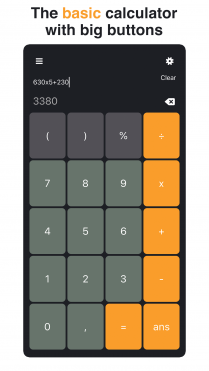•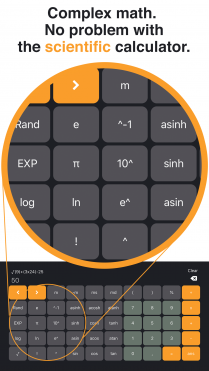•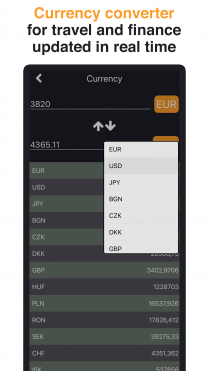•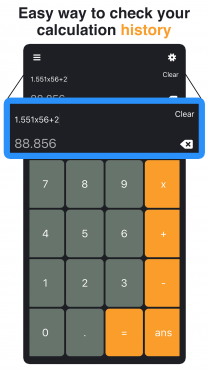•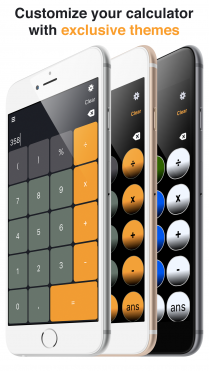•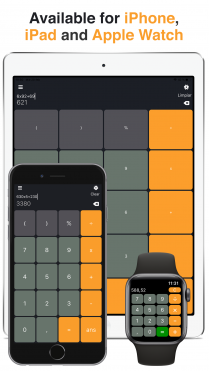5 stars on App Store

CalculatorApp Store5

### Calculator for iPhone, iPad and Apple Watch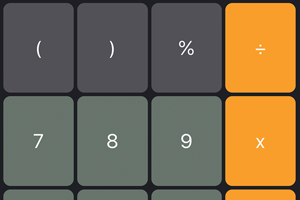### For Minecraft PE & PC

Calculator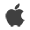Main features:

* Calculator 7 in 1
* Basic, scientific, converters for Currency, Distance, Weight, Time and Data
* Choose between the Basic or Scientific mode just by rotating your device
* Operate using degrees or radians
* Edit ecuations
* Memory bank
* Themes to customize your calculator as you wish
* intuitive and exclusive design

EDIT EQUATION
You can edit as many digits as the ones from the current equation.
You can drag the cursor with your finger.

LIST OF MATHEMATICAL OPERATIONS
Addition, subtraction, division, multiplication and percentages
Powers and roots
Scientific notation
Power of e, powers of base 10 and inverse powers
Neperian and decimal logarithms
Sine, cosine and tangent
Secant, cosecant, cotangent
Hyperbolic sine, hyperbolic cosine, hyperbolic and tangent
Inverse function, introduction of a random or factorial number

Calculator